Scientific Investigations Report 2007–5005

U.S. GEOLOGICAL SURVEY
Scientific Investigations Report 2007–5005

# Pond Discharge Equations

The structures used to control discharge from the ponds are culverts and weir boxes. Each pond has different structures (table 1).

A culvert is a conduit that allows water to flow between two bodies of water. All culverts in this study are round, 48-in. diameter, poly-coated, corrugated, metal pipes installed with no slope (that is, level) (fig. 2). Each culvert is inside a levee that separates the two water bodies. A discharge structure may include several culverts. Because the slough is tidal, its water level rises and falls by 3–10 ft during a tidal cycle. Flow is from the pond to the tidal slough when the water-surface elevation in the pond is higher than in the slough. A hinged flap gate on the slough side of each culvert prevents flow from entering the pond by closing when the water level in the slough rises above that in the pond. The flap gate is pushed open when the water level in the pond is greater than that in the slough and also can be raised or lowered manually to allow water to flow in both directions. A screw gate on the pond side of each culvert can be raised or lowered to an open position, partially open, or closed. A trash rack surrounds the discharge structure at the slough side of the culvert to prevent debris from clogging the culvert.

The other type of discharge structure present for some Alviso ponds is a weir box (fig. 3). The weir boxes are roughly 9 ft × 9 ft × 6 ft and made of cast concrete with six bays of removable wooden weir boards. Each bay functions as a sharp-crested, rectangular weir, and water discharges over the boards and enters a 48-in. culvert, which carries the water to the slough. Culverts associated with the weirs are the same as the culverts described above. For our calculations, we assume that the weir box typically controls discharge unless it is submerged or all of the weir boards are removed. The culvert will control discharge under these conditions.

## Theory

A simple culvert discharge equation can be derived by applying Bernoulli’s equation between the pond and slough sides of the culvert, by assuming that the water velocity at the trash rack is the same as the velocity in the culvert, and that the culvert is flowing full (Jobson and Froehlich, 1988). One form of the derived equation can be expressed as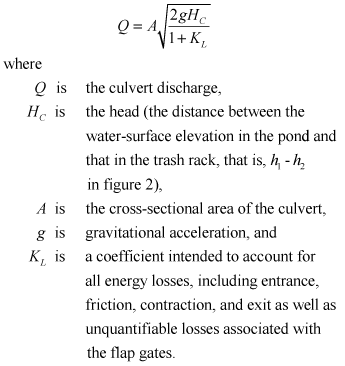(1)

The assumption that the culvert is flowing full is key to use of equation 1. During site investigations, pond culverts were observed to be flowing nearly full on the upstream ends (there was a gap of several inches below the crown of the culvert and the water surface), while the flap gates on the culvert outlets tended to create backwater in the culvert. The screw gates were fully opened. However, these conditions are not sufficient to maintain a full flowing culvert, so this assumption may not be valid. For this study, the loss coefficient, KL, was determined empirically from measurements of discharge from pond A3W, as is discussed in the next section of this report.

Weir discharge can be determined from a standard weir equation (Sturm, 2001) as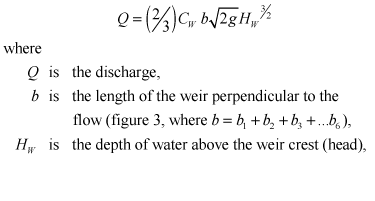(2)

and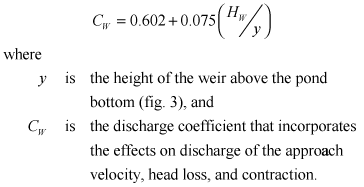(3)

## Measurements of Culvert Discharge

The culvert loss term, KL, was determined empirically from measurements of discharge from pond A3W. An acoustic Doppler current profiler (ADCP) (R.D. Instruments, 1,200 kHz, water mode five with 5 pings per second) was used to measure the discharge as described by Simpson (2002) and Oberg and others (2005). The ADCP emits an acoustic pulse, measures the return signal, then applies the principles of the Doppler shift to determine the velocity profile. The ADCP was mounted on a sled that floats on the water surface (fig. 4). A bottom-tracking feature allows the ADCP to determine its relative position as it moves along a path in the water. The discharge through the cross-sectional flow area below the path of the ADCP can be calculated using the measured velocity profile, bottom tracking, and water-surface elevation data. The sled in this study was towed with ropes from shore in a straight path on the pond side of the discharge structure about 12 ft upstream from the culvert intake. This location was chosen to ensure that water velocities would be large enough for reliable measurement, and the sled would be upstream and clear of the rapidly accelerating flow at the culvert intake (fig. 4). Measurements were collected upstream from the culvert because flow downstream from the culvert is highly non-uniform and is likely to introduce greater uncertainty in the measured discharge.

Measurements were collected at the pond A3W discharge structure on May 10, 2005. Pond A3W was selected for measurements because low dissolved oxygen concentrations in this pond dictated estimates of discharge as a requirement of the discharge permit granted to USFWS. Discharge and water-surface elevations were measured at intervals of 2–10 minutes from 07:59 to 08:39 a.m. and from 10:12 a.m. to 12:47 p.m. (all times given are in Pacific Standard Time). The measurement gap between 08:40 and 10:11 a.m. is due to equipment failure. The tide in the receiving water in Guadalupe Slough varied slightly from the water-surface elevation measured at the trash rack. Because the discharge point and trash rack are at a higher elevation than the bottom of the slough, during low tide the water surface in the trash rack is perched above the water surface in the slough. Therefore, the tide in the slough could be rising while the water-surface elevation in the trash rack is falling. Tidal predictions indicate that the tide was falling until roughly 08:30 a.m., while the water-surface elevation measured in the trash rack continued to drop until after 08:40 a.m. Water-surface elevations in the pond and the trash rack were measured from staff plates with marked elevations referenced to the National Geodetic Vertical Datum of 1929 (NGVD 29). The measured total discharge was divided by three to determine the discharge through a single culvert. Discharge through a single culvert, as a function of head (HC, the water-surface elevation difference), is shown in figure 5.

## Empirical Discharge Relations

Given measured pairs of head and discharge, equation 1 was solved to estimate KL. Ordinary least squares regression analysis was used to determine the best-fit relation between KL and head. The results indicate that the behavior of the culvert loss term is best described by two separate equations (fig. 6). At low heads (HC <0.63 ft), KL decreases exponentially with increasing head,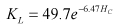(4)

The rapid decrease in KL probably is due to opening of the flap gate as head increases. At greater heads (HC <0.63 ft), KL increases linearly with an increase in head.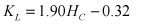(5)

The exponential function defined by equation 4 and the line defined by equation 5 intersect at HC = 0.63 ft. Equations 1, 4, and 5 provide the discharge-head relations for culverts used in PONDCALC. The measured and predicted single culvert discharges for pond A3W are compared in figure 5. Figure 5 indicates that the measured discharges closely match the predicted discharges for low heads (HC <0.63 ft), but measured discharges for high heads (HC >0.63 ft) have considerable scatter around the predicted discharges. The simple culvert discharge equation 1 evidently does not account fully for the more complex energy losses when heads are high. Nevertheless, the comparison shown in figure 5 indicates that PONDCALC estimates for culvert discharge are reasonable, overall. The discharge relation calculated from measured data obtained at pond A3W is assumed valid for the other discharge ponds in the Alviso Salt Pond Complex because their discharge structures are similar to that of pond A3W.

PONDCALC uses a standard weir equation (eq. 2) to estimate discharge through the weir box discharge structures. The head term in equation 2 does not include the water-surface elevation downstream from the weir; therefore, the reduction of discharge as the weir is submerged at higher tides is not simulated by the equation. To overcome this limitation with the weir equation, the culvert discharge also is calculated by PONDCALC. The calculated culvert discharge, which is based on the difference between pond and trash rack water-surface elevations (culvert head, HC) is dependant on tidal stage. The discharges calculated from the weir and culvert equations are compared, and the lower of the two values is the estimated discharge provided by PONDCALC. The lower value is used on the basis of the assumption that the weir box controls discharge when downstream water-surface elevations are low (low tide), and the culvert controls discharge when downstream water-surface elevations are high (high tide) and the weir is submerged.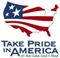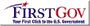U.S. Department of the Interior | U.S. Geological Survey